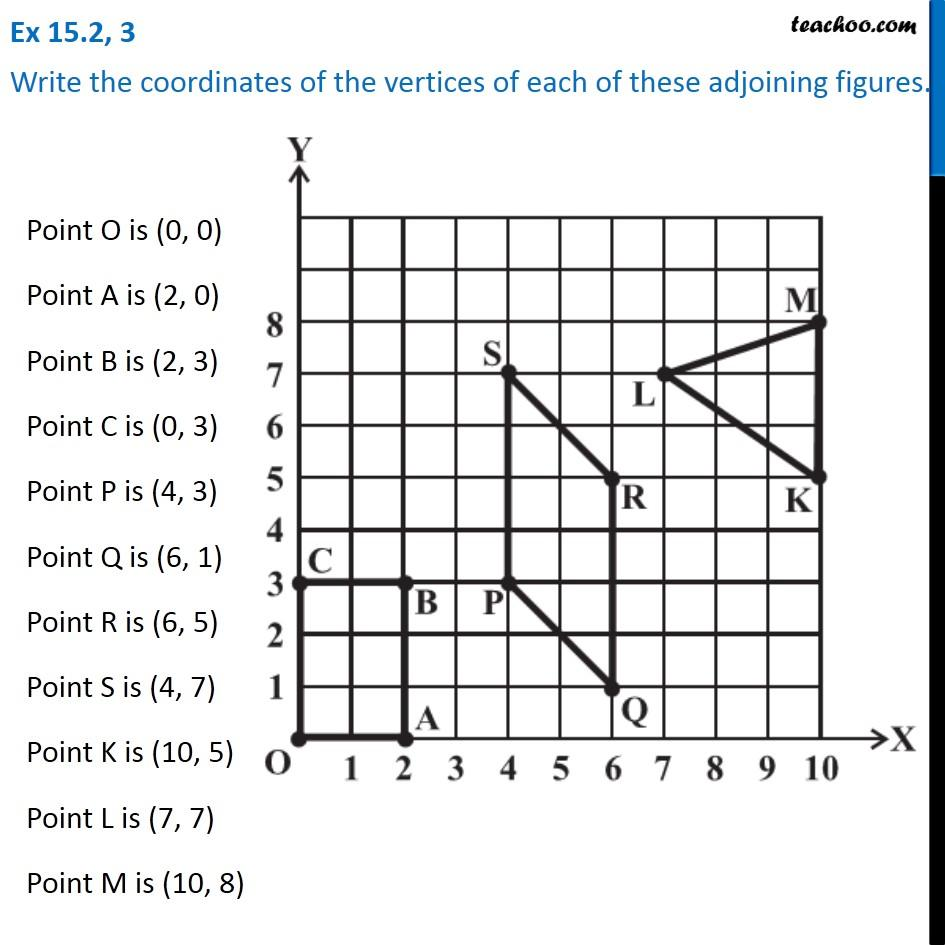Coordinates in a graph

Chapter 13 Class 8 Introduction to Graphs
Serial order wiseLearn in your speed, with individual attention - Teachoo Maths 1-on-1 Class

### Transcript

Question 3 Write the coordinates of the vertices of each of these adjoining figures.Point O is (0, 0) Point A is (2, 0) Point B is (2, 3) Point C is (0, 3) Point P is (4, 3) Point Q is (6, 1) Point R is (6, 5) Point S is (4, 7) Point K is (10, 5) Point L is (7, 7) Point M is (10, 8)